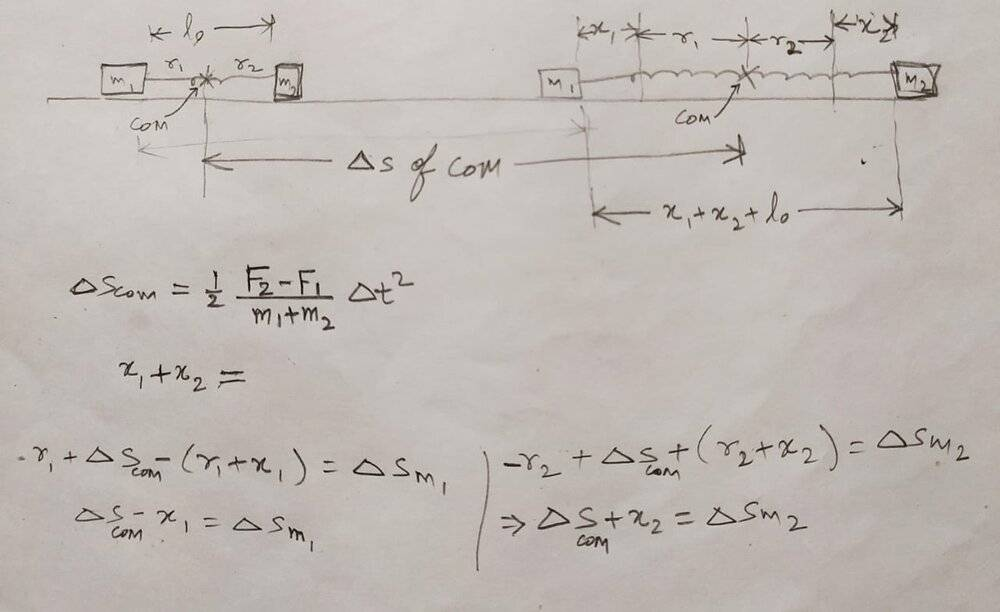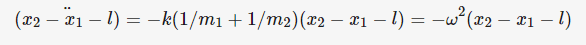# 2 Masses connected to a spring

@haruspex,
I've written the momentum equation:
$$\left ( F_2-F_1 \right)\Delta t=\left ( m_1+m_2 \right )v_{com}=m_1v_1+m_2v_2$$
and Energy equation :
$$F_1x_1+F_2x_2=\frac{1}{2}m_1v_1^{2}+\frac{1}{2}m_2v_2^{2}+\frac{1}{2}k\left ( x_1+x_2 \right )^{2}$$

But if I write another equation involving displacement and time, like following:
$$\Delta s=\frac{1}{2}\left ( \frac{F_2-F_1}{m_1+m_2} \right )\Delta t^2$$
then I'm including one more variable ##\Delta s##.
I am not able to write an equation involving displacement and time which doesn't involve a new variable. Where am I going wrong. Please help.

anuttarasammyak
Gold Member
Hi.
$$m_1 \ddot{x_1}=k(x_2-x_1-l_0)+F_1$$
$$m_2 \ddot{x_2}=-k(x_2-x_1-l_0)+F_2$$

#### Attachments

•img20201128_23304581.jpg
75.2 KB · Views: 37
•NTesla
@anuttarasammyak, Ok, now I understand those 2 equations. Thank you.
But, I still have one question: In the following equation that you've written in post#4:
$$\ddot{(x_2-x_1-l)}=-k(1/m_1+1/m_2)(x_2-x_1-l)=-\omega^2 (x_2-x_1-l)$$
##l##= total length of the string at any instant t=##x_2-x_1-l_0##
Therefore, ##(\ddot{x_2-x_1-l})##=##(\ddot{x_2-x_1-(x_2-x_1-l_0)})##=##(\ddot{-2x_1+l_0})##. This doesn't make sense to me. Please explain this particular equation that you've written.

haruspex
Homework Helper
Gold Member
2020 Award
then I'm including one more variable Δs.
That's the displacement of the mass centre. How does that relate to m1, m2, x1 and x2?

anuttarasammyak
Gold Member
@anuttarasammyak,
(x2−x1−l)¨=−k(1/m1+1/m2)(x2−x1−l)=−ω2(x2−x1−l)
l= total length of the string at any instant t=x2−x1−l0
Therefore, (x2−x1−l¨)=(x2−x1−(x2−x1−l0)¨)=(−2x1+l0¨). This doesn't make sense to me. Please explain this particular equation that you've written.
$$\ddot{(x_2-x_1)}=-k(1/m_1+1/m_2)(x_2-x_1-l_0)+F_2/m_2-F_1/m_1$$

You see RHS second term ##F_2/m_2-F_1/m_1## is constant.

You see RHS first term is written as
$$-k(1/m_1+1/m_2)(x_2-x_1-l_0)=-k(1/m_1+1/m_2)(x_2-x_1) - k(1/m_1+1/m_2)(-l_0)$$
the second term is constant.
Add these two constants and express it as
$$k(1/m_1+1/m_2)l$$
introducing new constant ##l##. Show me expression of ##l## by F,m,k and l_0.

Last edited:
@haruspex ,
I've tried to find a relation between CoM's displacement and ##x_1## and ##x_2##, but it appears more difficult than I assumed it to be. Here's my work:I am not able to figure out the relation. let me know how to proceed.

$$\ddot{(x_2-x_1)}=-k(1/m_1+1/m_2)(x_2-x_1-l_0)+F_2/m_2-F_1/m_1$$

You see RHS second term ##F_2/m_2-F_1/m_1## is constant.

You see RHS first term is written as
$$-k(1/m_1+1/m_2)(x_2-x_1-l_0)=-k(1/m_1+1/m_2)(x_2-x_1) - k(1/m_1+1/m_2)(-l_0)$$
the second term is constant.
Add these two constants and express it as
$$k(1/m_1+1/m_2)l$$
introducing new constant ##l##. Show me expression of ##l## by F,m,k and l_0.
@anuttarasammyak, Perhaps I hadn't made the question clear in my earlier post. I do understand the calculation part of it. What I couldn't understand is that what is the physical significance of the variable which you have double differentiated in the equation:anuttarasammyak
Gold Member
What I couldn't understand is that what is the physical significance of the variable which you have double differentiated in the equation:
You observe that this is equation of harmonic oscillation : displacement ##x_2-x_1-l##, angular frequency ##\omega##.

l is new "natural length" of spring with ##F_1## and ##F_2## applied. ##x_2-x_1-l## is extension of spring from renewed balance point. See also HINT in my previous post.

Last edited:
You observe that this is equation of harmonic oscillation : displacement ##x_2-x_1-l##, angular frequency ##\omega##.

l is new "natural length" of spring with ##F_1## and ##F_2## applied. ##x_2-x_1-l## is extension of spring from renewed balance point. See also HINT in my previous post.
@anuttarasammyak,
Since ##x_1, x_2## are coordinates of masses at any instant.
##l##=##x_2-x_1##. Therefore, ##x_2-x_1-l## = ##x_2-x_1-x_2+x_1## = ##0## always. So there is no reason why this should be differentiated wrt time.

Last edited:
anuttarasammyak
Gold Member
Since ##x_1, x_2## are coordinates of masses at any instant.
##l##=##x_2-x_1##. Therefore, ##x_2-x_1-l## = ##x_2-x_1-x_2+x_1## = ##0## always. So there is no reason why this should be differentiated wrt time.
You have done the calculation so know that ##l## is constant. So your ##l##=##x_2-x_1## means spring between block 1 and block 2 does not change its length like a rod, though the equation is that of harmonic oscillation. Do I catch you right ?

You have done the calculation so know that ##l## is constant. So your ##l##=##x_2-x_1## means spring between block 1 and block 2 does not change its length like a rod, though the equation is that of harmonic oscillation. Do I catch you right ?
I'm trying to understand your calculation in post #4. You have taken ##x_1,x_2## as coordinates.
##l## is the length of string at any instant of time. It is not a constant. ##l## changes wrt time.
##l##=##x_2-x_1##, considering that ##x_1, x_2## are coordinates of the masses ##m_1## and ##m_2##.
But, the value of ##x_2-x_1-l=0## always.

Last edited:
anuttarasammyak
Gold Member
Hi. My l is
$$l-l_0=\frac{F_2/m_2-F_1/m_1}{\omega^2}$$

It is not a constant. ##l## changes wrt time.
##l##=##x_2-x_1##, considering that ##x_1, x_2## are coordinates of the masses ##m_1## and ##m_2##.
But, the value of ##x_2-x_1-l=0## always.

So the equation is written as
$$\ddot{(l_{yours}-l_{mine})}+\omega^2(l_{yours}-l_{mine})=0$$
where
$$\omega^2=k(1/m_1+1/m_2)$$
$$l_{mine}=l_0+\frac{F_2/m_2-F_1/m_1}{\omega^2}$$, constant, and
$$l_{yours}=x_2-x_1=l_{yours}(t)$$ function of time.

##l_{yours}-l_{mine}## is elongation of spring not from its natural length ##l_0## but from ##l_{mine}## which is interpreted as altered natural length under external constant forces.

Last edited:
haruspex
Homework Helper
Gold Member
2020 Award
@haruspex ,
I've tried to find a relation between CoM's displacement and ##x_1## and ##x_2##, but it appears more difficult than I assumed it to be. Here's my work: View attachment 273350

I am not able to figure out the relation. let me know how to proceed.
If m1 moves x1 to the left and m2 moves x2 to the right then that shifts the "mass moment" m2 x2 - m1 x1 to the right, so (m1+m2)Δs = m2 x2 - m1 x1, where Δs is positive to the right.

@haruspex, Thank you so much. That was very helpful.

Just one question: If we do not resort to center of mass FoR, and work solely in Ground FoR, then how can we explain that the maximum elongation will happen when both the masses have same velocity as that of velocity of com ?

haruspex
Homework Helper
Gold Member
2020 Award
@haruspex, Thank you so much. That was very helpful.

Just one question: If we do not resort to center of mass FoR, and work solely in Ground FoR, then how can we explain that the maximum elongation will happen when both the masses have same velocity as that of velocity of com ?
Not sure if that's what you meant. There's no problem explaining that; do you mean, how do we use it?
Isn't it the same as saying the two masses have the same velocity?

@haruspex,
When working in CoM FoR, both the masses will seem to be moving in opposite direction and maximum extension in spring will happen when velocity of both the masses is equal to zero at the same instant.

However, when working in Ground FoR, the bodies might seem to be moving in same direction, but maximum extension of spring will happen when both the masses have same speed in same direction. Will it be right to say so.? If yes, then is there an intuitive way to explain that this is the only way possible for string to have maximum elongation in Ground FoR ?

anuttarasammyak
Gold Member
Hi.
If yes, then is there an intuitive way to explain that this is the only way possible for string to have maximum elongation in Ground FoR ?
At extreme points
$$\dot{l_{yours}}=0$$
It means
$$\dot{x_1}=\dot{x_2}$$
When it is maximum extension
$$\ddot{l_{yours}}=-\omega^2(l_{yours}-l_{mine})< 0$$
For minimum shrink
$$\ddot{l_{yours}}=-\omega^2(l_{yours}-l_{mine})> 0$$

•NTesla
haruspex
•